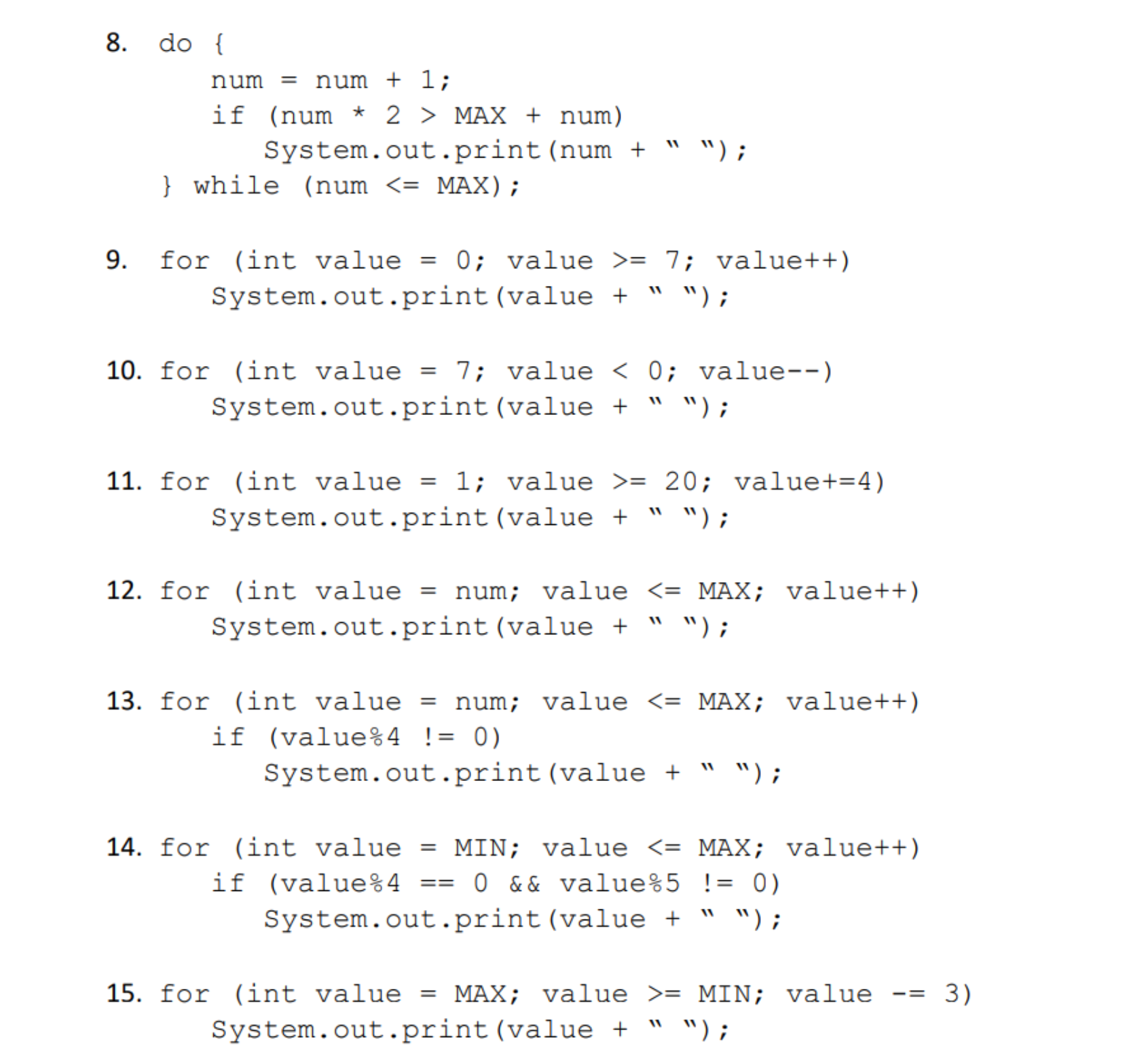# 8. do[num = num + 1 ;if (num * 2 〉 MAX + num)System.out.print (num + "");while (num - 7; value++)System.out.print (value " ");10. f r (int value = 7: value < 0; value-_)System. out.print (value + " w);11. for (int value - 1; value -20; value+-4)System.out.print (value + " W);12. for (int value -num; valueMAX; value++System.out.print (value " ");13. for (int value - num; valueMAX; value++)System.out.print (value " ");14. for (int value-MIN; value 〈-MAX ; value++)System.out.print (value " ");if(value%4!0)15. f r (int value = MAX ; value 〉= MIN; value-= 3)System. out.print (value + " w);

Question
48 views

Assume the following declarations and initializations (MIN, MAX, num) are made just before each sub-question 1 to 15. What is the output each code?help_outlineImage Transcriptionclose8. do[ num = num + 1 ; if (num * 2 〉 MAX + num) System.out.print (num + ""); while (num <- MAX) 9. for (int value0 value >- 7; value++) System.out.print (value " "); 10. f r (int value = 7: value < 0; value-_) System. out.print (value + " w); 11. for (int value - 1; value -20; value+-4) System.out.print (value + " W); 12. for (int value -num; valueMAX; value++ System.out.print (value " "); 13. for (int value - num; valueMAX; value++) System.out.print (value " "); 14. for (int value-MIN; value 〈-MAX ; value++) System.out.print (value " "); if (value%4 ! 0) 15. f r (int value = MAX ; value 〉= MIN; value-= 3) System. out.print (value + " w); fullscreen
check_circle

Step 1

Since these are separate blocks of code, for the purpose of demonstration we put the blocks in same Java class and show the execution output together. The questions taken up are from 8 to 15 as given. Also since the initializations of MIN, MAX, num are not given we assume suitable values by passing command line arguments. The program for the demonstration is given below:

class PrintDemo{

public static void main(String args[]){

//run program using 3 command line arguments for num, //MIN, MAX

int num = Integer.parseInt(args);

int MIN = Integer.parseInt(args);

int MAX = Integer.parseInt(args);

System.out.println("---------Q8----------");

do{

num = num +1;

if(num * 2 > MAX + num)

System.out.print(num + " ");

}while(num <= MAX);

System.out.println("\n---------Q9----------");

for(int value=0; value >= 7; value++)

System.out.print(value + " ");

System.out.println("\n---------Q10----------");

for(int value=7; value < 0; value--)

System.out.print(value + " ");

Sy...

### Want to see the full answer?

See Solution

#### Want to see this answer and more?

Solutions are written by subject experts who are available 24/7. Questions are typically answered within 1 hour.*

See Solution
*Response times may vary by subject and question.
Tagged in

### Computer Science